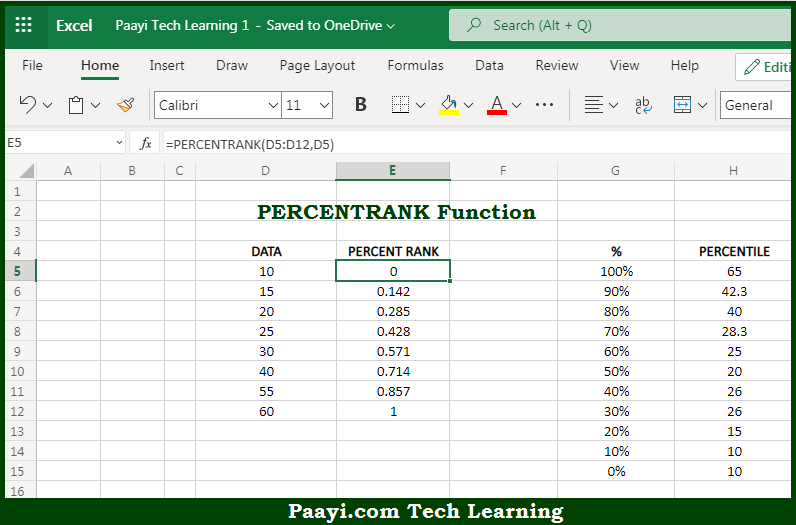# Learn How to Use Microsoft Excel PERCENTRANK Function

Written by | 0 Comments | 635 Views

In this article, you will learn how to use the Microsoft Excel PERCENTRANK function and its prime function in Microsoft Excel. You will also get to know the Microsoft Excel PERCENTRANK function return value and syntax with the help of some examples.

Microsoft Excel PERCENTRANK Function

The main purpose of the Microsoft Excel PERCENTRANK function is to get the percentile rank, inclusive. That implies, with the help of the PERCENTRANK function you can able to return the rank of a value in a given data set as a percentage of the data set. It should be noted that the PERCENTRANK function can be used to find the relative standing of a value within a data set. The percentile rank is commonly used as a way to interpret standing in standardized tests. So, with the help of the PERCENTRANK function, you can able to get the percentile rank, inclusive.

Return Value of PERCENTRANK Function

The return value will be the calculated rank in the decimal value.

Syntax of PERCENTRANK Function

=PERCENTRANK(array, x, [significance])

Where the arguments:

• array: This is the data value array.
• x: This is the value to rank.
• significance: This is the number of significant digits in the result. Defaults to 3 (optional).

## How to Use Microsoft Excel PERCENTRANK Function?So we know that Microsoft Excel PERCENTRANK function you can able to get the percentile rank, inclusive. That implies, with the help of the PERCENTRANK function you can able to return the rank of a value in a given data set as a percentage of the data set. It should be noted that the PERCENTRANK function can be used to find the relative standing of a value within a data set. The percentile rank is commonly used as a way to interpret standing in standardized tests. So, with the help of the PERCENTRANK function, you can able to get the percentile rank, inclusive.